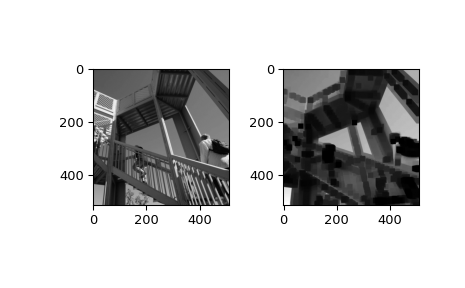# scipy.ndimage.minimum_filter¶

scipy.ndimage.minimum_filter(input, size=None, footprint=None, output=None, mode='reflect', cval=0.0, origin=0)[source]

Calculate a multidimensional minimum filter.

Parameters
inputarray_like

The input array.

sizescalar or tuple, optional

See footprint, below. Ignored if footprint is given.

footprintarray, optional

Either size or footprint must be defined. size gives the shape that is taken from the input array, at every element position, to define the input to the filter function. footprint is a boolean array that specifies (implicitly) a shape, but also which of the elements within this shape will get passed to the filter function. Thus `size=(n,m)` is equivalent to `footprint=np.ones((n,m))`. We adjust size to the number of dimensions of the input array, so that, if the input array is shape (10,10,10), and size is 2, then the actual size used is (2,2,2). When footprint is given, size is ignored.

outputarray or dtype, optional

The array in which to place the output, or the dtype of the returned array. By default an array of the same dtype as input will be created.

modestr or sequence, optional

The mode parameter determines how the input array is extended when the filter overlaps a border. By passing a sequence of modes with length equal to the number of dimensions of the input array, different modes can be specified along each axis. Default value is ‘reflect’. The valid values and their behavior is as follows:

‘reflect’ (d c b a | a b c d | d c b a)

The input is extended by reflecting about the edge of the last pixel. This mode is also sometimes referred to as half-sample symmetric.

‘constant’ (k k k k | a b c d | k k k k)

The input is extended by filling all values beyond the edge with the same constant value, defined by the cval parameter.

‘nearest’ (a a a a | a b c d | d d d d)

The input is extended by replicating the last pixel.

‘mirror’ (d c b | a b c d | c b a)

The input is extended by reflecting about the center of the last pixel. This mode is also sometimes referred to as whole-sample symmetric.

‘wrap’ (a b c d | a b c d | a b c d)

The input is extended by wrapping around to the opposite edge.

For consistency with the interpolation functions, the following mode names can also be used:

‘grid-constant’

This is a synonym for ‘constant’.

‘grid-mirror’

This is a synonym for ‘reflect’.

‘grid-wrap’

This is a synonym for ‘wrap’.

cvalscalar, optional

Value to fill past edges of input if mode is ‘constant’. Default is 0.0.

originint or sequence, optional

Controls the placement of the filter on the input array’s pixels. A value of 0 (the default) centers the filter over the pixel, with positive values shifting the filter to the left, and negative ones to the right. By passing a sequence of origins with length equal to the number of dimensions of the input array, different shifts can be specified along each axis.

Returns
minimum_filterndarray

Filtered array. Has the same shape as input.

Notes

A sequence of modes (one per axis) is only supported when the footprint is separable. Otherwise, a single mode string must be provided.

Examples

```>>> from scipy import ndimage, misc
>>> import matplotlib.pyplot as plt
>>> fig = plt.figure()
>>> plt.gray()  # show the filtered result in grayscale
>>> ax1 = fig.add_subplot(121)  # left side
>>> ax2 = fig.add_subplot(122)  # right side
>>> ascent = misc.ascent()
>>> result = ndimage.minimum_filter(ascent, size=20)
>>> ax1.imshow(ascent)
>>> ax2.imshow(result)
>>> plt.show()
```